# Test: Properties Of Vectors

## 10 Questions MCQ Test Mathematics (Maths) Class 12 | Test: Properties Of Vectors

Description
This mock test of Test: Properties Of Vectors for JEE helps you for every JEE entrance exam. This contains 10 Multiple Choice Questions for JEE Test: Properties Of Vectors (mcq) to study with solutions a complete question bank. The solved questions answers in this Test: Properties Of Vectors quiz give you a good mix of easy questions and tough questions. JEE students definitely take this Test: Properties Of Vectors exercise for a better result in the exam. You can find other Test: Properties Of Vectors extra questions, long questions & short questions for JEE on EduRev as well by searching above.
QUESTION: 1

### Direction of the resultant of two vectors is given by

Solution:

Assume the two vector A and B with an angle alpha whose resultant be vector C given by vector C = vector A + vector B. In addition drop a line from the point B and C meeting to form the right angled triangle, so AC2 = AD2 + CD2
⇒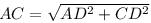⇒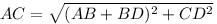⇒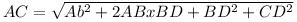⇒ From triangle BCD, cos alpha = BD / BC
⇒ BD = BC x cos∝
⇒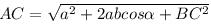⇒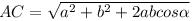The resultant vector makes angle theta therefore, in triangle BCD,
sin∝ = CD/BC
⇒ CD = BCsinα,
tanθ = CD/AD = BC sinα/a + bcos∝
⇒ θ = tan -1 bsin∝/a + bcosα

QUESTION: 2

### The position vector of mid-point of joining the points (2, – 1, 3) and (4, 3, –5) is :

Solution:

The position vector of point P = 2i - j + 3k
Position Vector of point Q = 4i + 3j - 5k
The position vector of R which divides PQ in half is given by:
r = (2i - j + 3k + 4i + 3j - 5k)/2
r = (6i + 2j - 2k)/2
r = 3i + j - k

QUESTION: 3

### The Position vector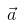of a point (12,n) is such that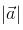= 13 then n = ​

Solution:

Position vector of a =12i+nj
|a|=√144+n^2=13
squaring
144+n^2=169
n^2=25
n=±5

QUESTION: 4

The vector joining the points A(2, – 3, 1) and B(1, – 2, – 5) directed from B to A is:

Solution:

Initial coordinates = i - 2j -5k
Final coordinates = 2i - 3j + k
Final - Initial = [ (2-1)i + (-3+2) j + (1+5)k ]
= i - j + 6k.

QUESTION: 5

ABCD is a parallelogram. If coordinates of A,B,C are (2,3), (1,4) and (0, -2). Coordinates of D =​

Solution:

As ABCD is a parallelogram, to find the fourth coordinate add the adjacent coordinate and then subtract opposite coordinate.
like D = A + C - B
= (2 + 0 - 1, 3 + (-2) -4)
= (1,-3)

QUESTION: 6

The position vectors of the end points of diameter of a circle are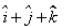and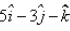, then the position vector of the centre of the circle is:

Solution:

{(1+5)î +(1-3)j + (1-1)k} / 2
= {6i - 2j + 0k}/2
= 3i - j

QUESTION: 7

If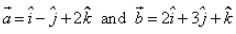, then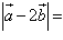........

Solution:

a= i-j+2k  b= 2i+3j+k
2b = 4i + 6j + 2k
|a - 2b| = (i - j + 2k) - (4i + 6j + 2k)
= -3i -7j + 0k
|a - 2b| = [(-3)2 + (-7)2 + (0)2]½
= [9 + 49]½
⇒ 1/2

QUESTION: 8

The points with position vectors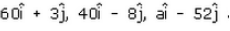are collinear vectors, Value of a =​

Solution:

Position vector A = 60i+3j
Position vector B = 40i-8j
Position vector C = aj-52j
Now, find vector AB and BC
AB = -20i-11j
BC= (a-40)i-44j
To be collinear,  angle between the vector AB and BC made by the given position vectors should be 0 or 180 degree.
That’s why the cross product of  the vectors should be zero
ABXBC=(-20i-11j)X(a-40)i-44j
0i+0j+(880+11(a-40))=0
a-40= -80
a=-40
Therefore, a should be -40 to be the given positions vectors collinear.

QUESTION: 9

The distance between the point (2, 3, 1) and (–1, 2, – 3) is:​

Solution:
QUESTION: 10

In the triangle ABC, which statement is not true?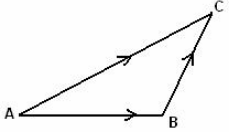Solution: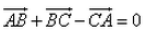is correct because the direction between the A&C is opposite, thats why negative sign is in between the BC and CA.